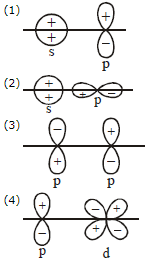Select the correct order of solubility (in water) from the following:

(1) SrSO4 < CaSO4 < MgSO4 < BeSO4

(2) NaF < KF < RbF < CsF

(3) Ba(OH)2 > Sr(OH)2 > Ca(OH)2 > Mg(OH)2

(4) All of these

Concept Questions :-

Lattice/Hydration Energy
High Yielding Test Series + Question Bank - NEET 2020

Difficulty Level:

Which of the following exhibits H-bonding?

1. CH4

2. H2Se

3. N2H4

4. H2S

Concept Questions :-

Hydrogen Bonding
High Yielding Test Series + Question Bank - NEET 2020

Difficulty Level:

Amongst  the one with highest boiling point is:

1. H2O because of H-bonding

2. H2Te because of higher molecular weight

3. H2S because of H-bonding

4. H2Se because of lower molecular weight

Concept Questions :-

Hydrogen Bonding
High Yielding Test Series + Question Bank - NEET 2020

Difficulty Level:

Which of the following leads to bonding?Concept Questions :-

Covalent Bonding
High Yielding Test Series + Question Bank - NEET 2020

Difficulty Level:

Which of the following are isoelectronic and isostructural?

(1)

(2)

(3)

(4)

Concept Questions :-

Types of Bonding
High Yielding Test Series + Question Bank - NEET 2020

Difficulty Level:

Which of the following has largest bond angle.

1. H2O

2. F2O

3. Cl2O

4. H2S

Concept Questions :-

M.O.T
High Yielding Test Series + Question Bank - NEET 2020

Difficulty Level:

In the molecular orbital diagram for ${\mathrm{O}}_{2}^{+}$ ion. the HOMO is :-

(1) $\sigma$ MO orbital

(2) π MO orbital

(3)  π* MO orbital

(4) $\stackrel{*}{\sigma }$ MO orbital

Concept Questions :-

M.O.T
High Yielding Test Series + Question Bank - NEET 2020

Difficulty Level:

Which of the following have a three dimensional network structure?

(1) SiO2

(2) (BN)y

(3) P4(white)

(4) 1 and 2 both

Concept Questions :-

Covalent Bonding
High Yielding Test Series + Question Bank - NEET 2020

Difficulty Level:

The correct order of strength of H-bond in the following compound :-

(1) H2O > H2O2 > HF > H2S

(2) HF >  H2O2 > H2O > H2S

(3) HF > H2O > H2S > H2O2

(4) HF > H2O > H2O2 > H2S

Concept Questions :-

Hydrogen Bonding
High Yielding Test Series + Question Bank - NEET 2020

Difficulty Level:

Which of the following pair of species have identical shapes ?

(1) NO2+ and NO2-

(2)PCl5 and BeF5

(3) XeF4 and ICI4-

(4) TeCl4 and XeO4

Concept Questions :-

Hybridisation